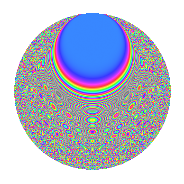# Properties

 Label 2015.2.mLevel 2015 Weight 2 Character orbit m Rep. character $$\chi_{2015}(993,\cdot)$$ Character field $$\Q(\zeta_{4})$$ Dimension 420 Sturm bound 448

# Related objects

## Defining parameters

 Level: $$N$$ = $$2015 = 5 \cdot 13 \cdot 31$$ Weight: $$k$$ = $$2$$ Character orbit: $$[\chi]$$ = 2015.m (of order $$4$$ and degree $$2$$) Character conductor: $$\operatorname{cond}(\chi)$$ = $$65$$ Character field: $$\Q(i)$$ Sturm bound: $$448$$

## Dimensions

The following table gives the dimensions of various subspaces of $$M_{2}(2015, [\chi])$$.

Total New Old
Modular forms 456 420 36
Cusp forms 440 420 20
Eisenstein series 16 0 16

## Trace form

 $$420q - 420q^{4} + 8q^{5} + O(q^{10})$$ $$420q - 420q^{4} + 8q^{5} - 16q^{11} + 16q^{12} - 24q^{13} - 8q^{15} + 420q^{16} - 28q^{17} - 52q^{18} - 8q^{19} - 20q^{20} + 8q^{21} + 16q^{22} + 32q^{23} + 32q^{24} + 20q^{25} - 32q^{26} + 20q^{34} + 56q^{37} - 8q^{39} - 96q^{40} - 4q^{41} + 16q^{43} + 24q^{44} - 40q^{45} - 8q^{46} - 32q^{48} + 356q^{49} - 52q^{50} + 36q^{52} + 12q^{53} - 32q^{54} - 64q^{55} - 40q^{58} - 8q^{59} + 76q^{60} - 420q^{64} - 48q^{65} - 44q^{68} + 32q^{69} + 152q^{70} - 24q^{71} + 156q^{72} - 32q^{75} - 8q^{76} + 32q^{77} - 24q^{78} + 120q^{80} - 404q^{81} - 52q^{82} - 24q^{83} + 40q^{84} - 100q^{85} + 104q^{86} + 32q^{87} + 32q^{88} - 36q^{89} - 84q^{90} - 16q^{91} - 96q^{92} + 64q^{95} - 168q^{96} + 24q^{99} + O(q^{100})$$

## Decomposition of $$S_{2}^{\mathrm{new}}(2015, [\chi])$$ into irreducible Hecke orbits

The newforms in this space have not yet been added to the LMFDB.

## Decomposition of $$S_{2}^{\mathrm{old}}(2015, [\chi])$$ into lower level spaces

$$S_{2}^{\mathrm{old}}(2015, [\chi]) \cong$$ $$S_{2}^{\mathrm{new}}(65, [\chi])$$$$^{\oplus 2}$$